Function Repository Resource:

# CurveToBSplineFunction

Create a smooth parametrized function from a list of points with flexible control over smoothing

Contributed by: Alexey Popkov
 ResourceFunction["CurveToBSplineFunction"][{pt1,pt2,…}, d] creates a B-spline function of maximal degree d parametrizing a curve defined by the points pti. ResourceFunction["CurveToBSplineFunction"][{pt1,pt2,…}, d, scale] specifies that the scale factor scale should be used for interval subdivision.

## Details and Options

ResourceFunction["CurveToBSplineFunction"] returns a BSplineFunction object.
Before processing, successive duplicate points are removed from the input list of points {pt1,pt2,}.
ResourceFunction["CurveToBSplineFunction"][{pt1,pt2,},d] is equivalent to ResourceFunction["CurveToBSplineFunction"][{pt1,pt2,},d,1].
The second argument d determines the maximal degree of the underlying polynomial basis used by a BSplineFunction object. Increasing this value leads to a smoother spline with fewer details.
The third argument scale is the scale factor used for filling the gaps between successive points of the curve when the option "FillGaps"True is set. With this setting,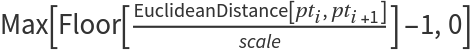equidistant control points will be introduced between the points pti and pti+1. By default, it is assumed that a scale of 1 means that no intermediate control points will be introduced when the Euclidean distance between successive points is less than 2. Increasing this value leads to a smoother spline with fewer details.
The following options can be given:
 "AssignWeights" False controls assigning weights to the control point of a generated spline "CurveClosed" False whether to treat the input curve defined by points {pt1,pt2,…} as closed "DuplicateNodes" False controls duplication of the input control points pti "FillGaps" True whether to introduce intermediate control points
The option setting "AssignWeights"Automatic assigns to every control point of a generated spline the weight equal to the sum of its distances to the two adjacent points. With the setting "AssignWeights"f, where f is a function, the weight of the point will be set to f[pti, {l, r}, angle, scale]. This option helps to preserve the shape of the original curve by selectively increasing the weights of the control points.
With the setting "CurveClosed"True, the input list of points {pt1,pt2,} is treated as cyclic.
The option "DuplicateNodes" gives flexible control over duplication of the input control points pti inside of a produced BSplineFunction. With the setting "DuplicateNodes"n, every point will be duplicated n times. With the setting "DuplicateNodes"Automatic, every input point pti will be duplicated Max[Floor[(l+r)/(2 scale)],1] times, where l and r are Euclidean distances to the adjacent points from the left and the right, respectively. With "DuplicateNodes"f, where f is a function, the point will be duplicated f[pti, {l, r}, angle, scale] times, where angle is the interior angle at the vertex point pti (for the boundary points, angle is set to Infinity). This option allows preservation of the sharp corners of the original curve by duplicating the corresponding control points.

## Examples

### Basic Examples (2)

Convert a 2D curve into a spline function preserving the original shape with different accuracy:

 In:=Out=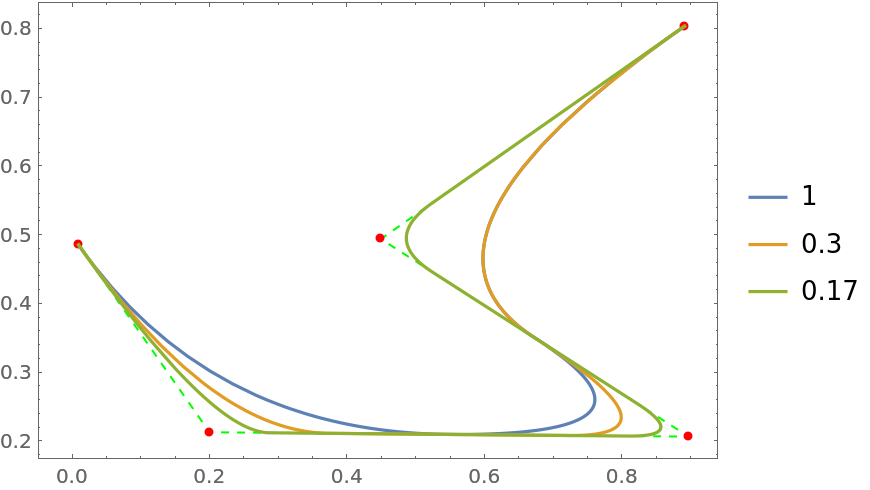Parametrize 3D data:

 In:=Out=Out=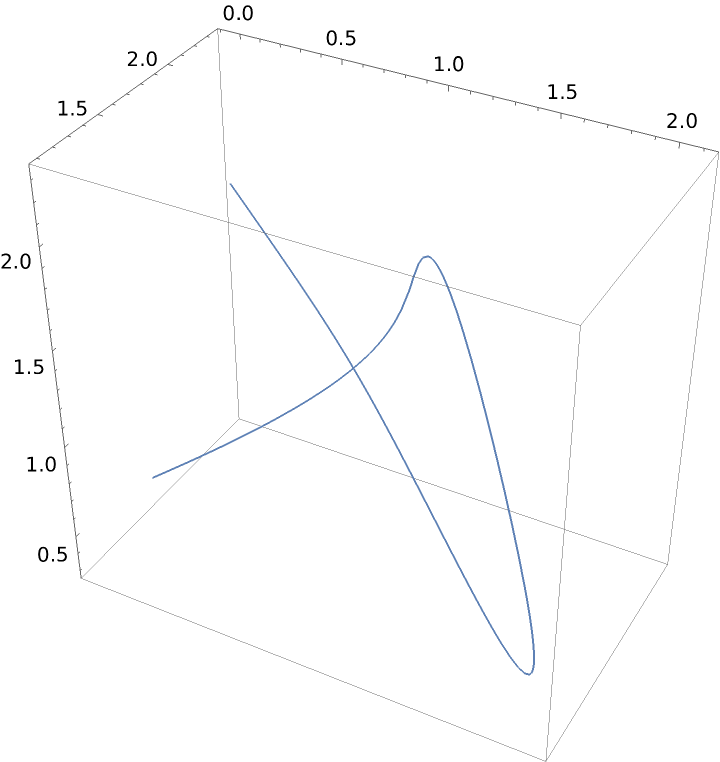### Scope (1)

Parametrization of an arbitrary polygon with different scale factors:

 In:=Out=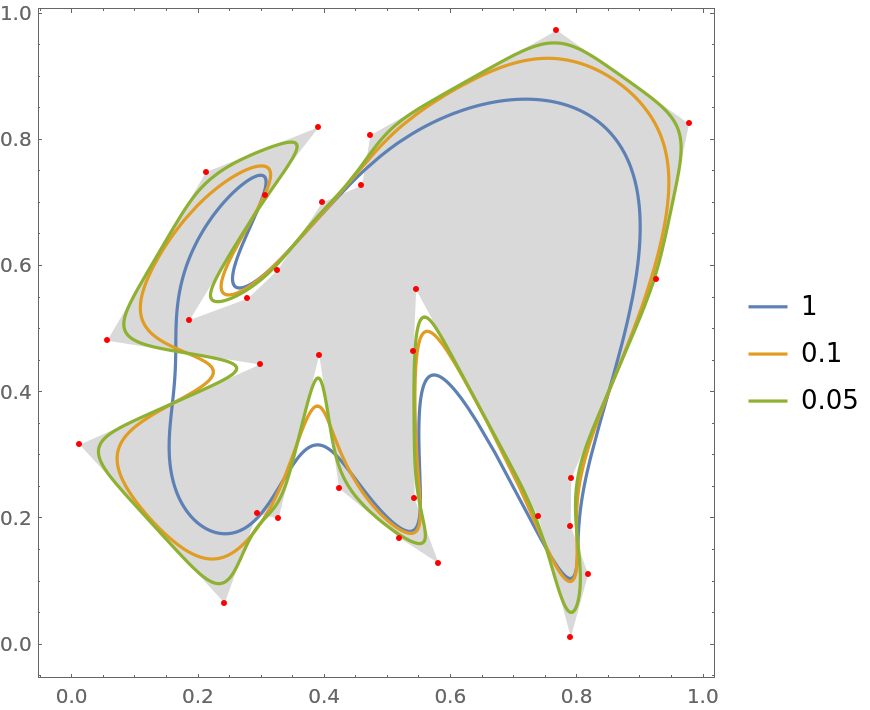### Options (7)

#### AssignWeights

With option value "AssignWeights"Automatic, original control points get weights equal to the sum of their Euclidean distances to the adjacent points, which is equivalent to "AssignWeights"(Total[#2]&). Compare with a weighting function dependent on the interior angle at the node (argument #3):

 In:=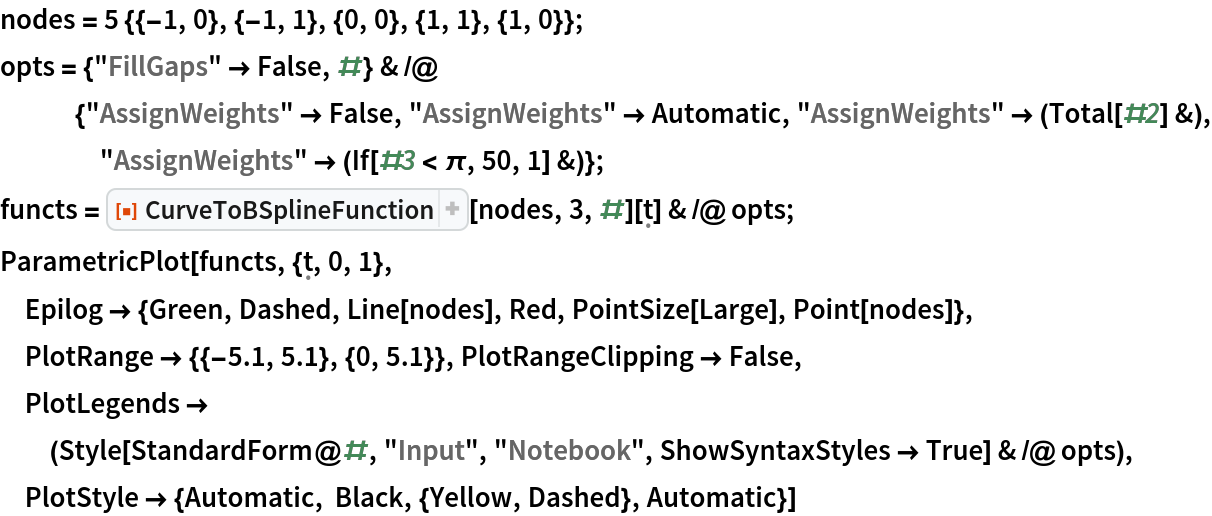Out=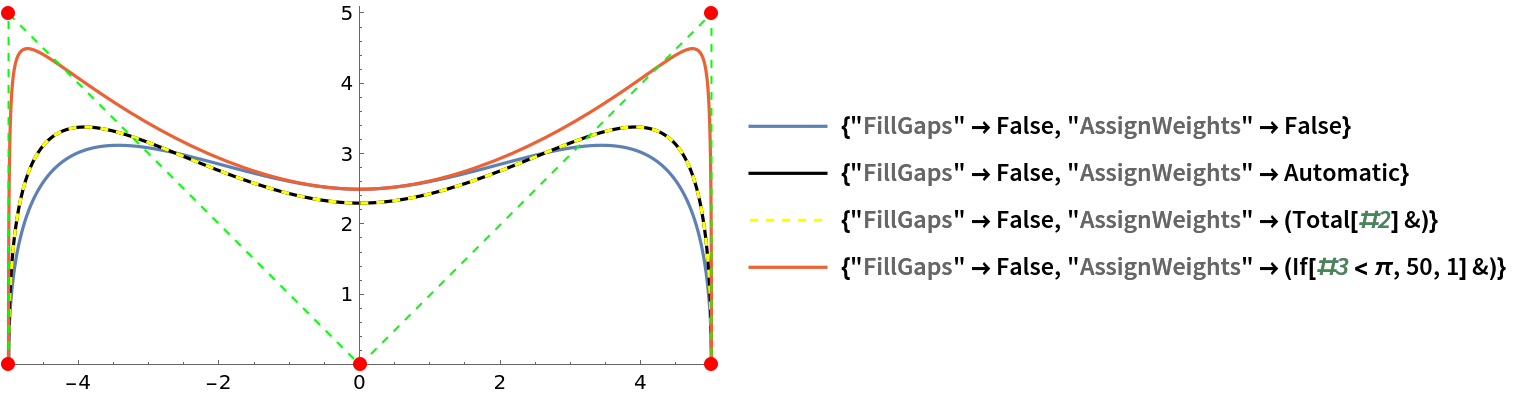#### CurveClosed

With option value "CurveClosed"True, a parametric function describing a closed contour is produced. Its geometric form is invariant to the rotation of the points:

 In:=Out=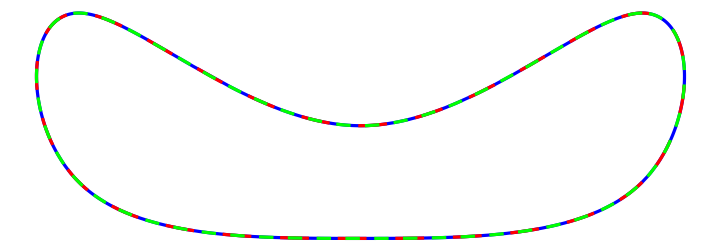#### DuplicateNodes

With option value "DuplicateNodes"n, original distinct control points will be duplicated n times. The default option value "DuplicateNodes"False is equivalent to "DuplicateNodes"1 (no point duplication). The option value "DuplicateNodes"Automatic tries to preserve significant sharp features of the original shape and is equivalent to "DuplicateNodes"(Max[Floor[Total[#2]/(2#4)],1]&). Compare them with a node duplication function dependent on the interior angle at the node (argument #3):

 In:=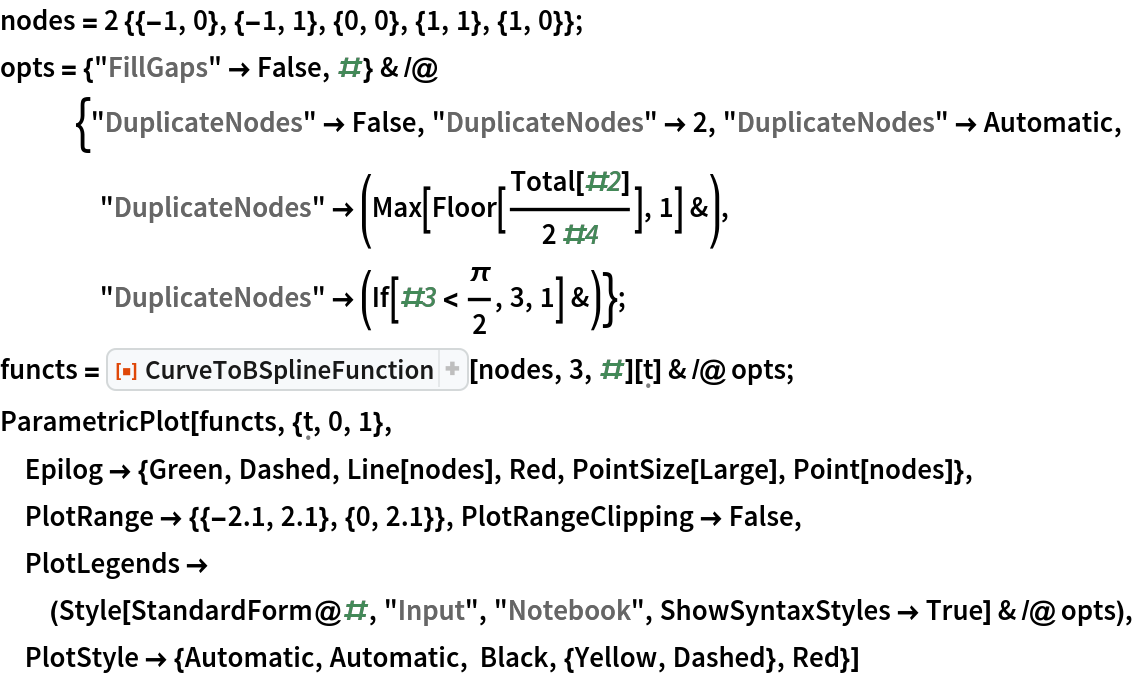Out=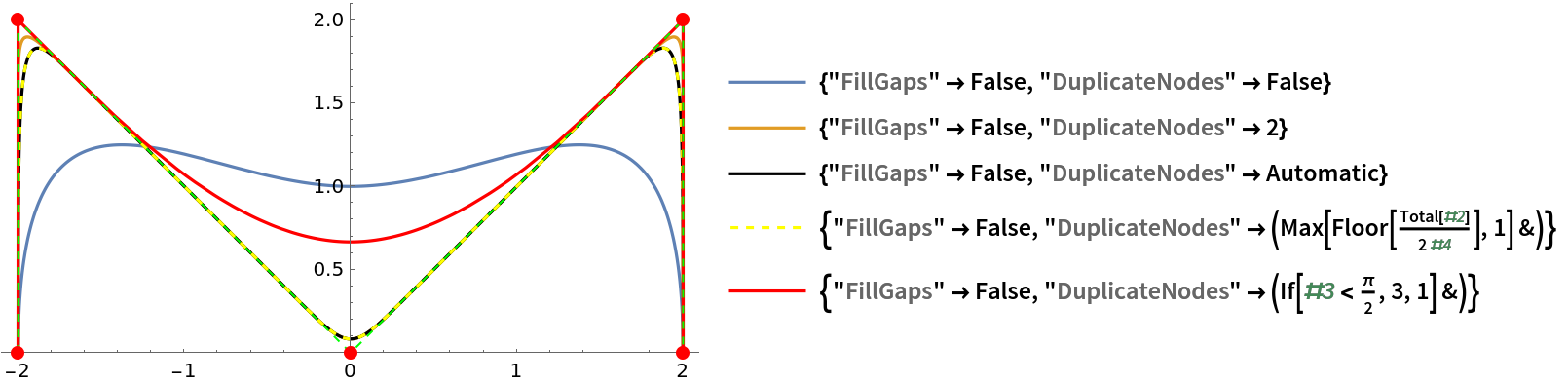#### FillGaps

With the default option value "FillGaps"True, intermediate control points are added when the distance between successive nodes is at least twice the scale:

 In:=Out=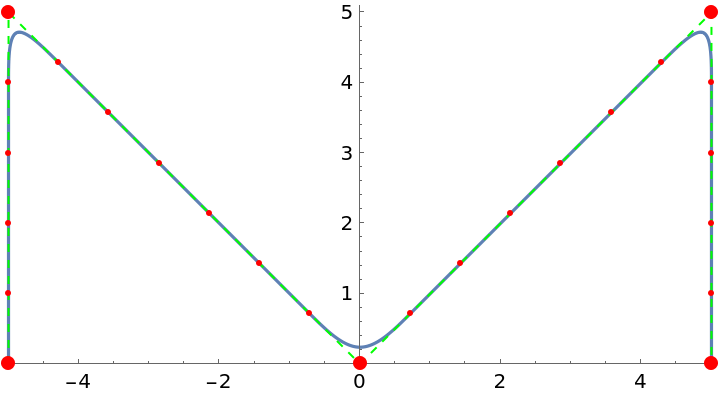Turn off introduction of intermediate control points by specifying "FillGaps"False:

 In:=Out=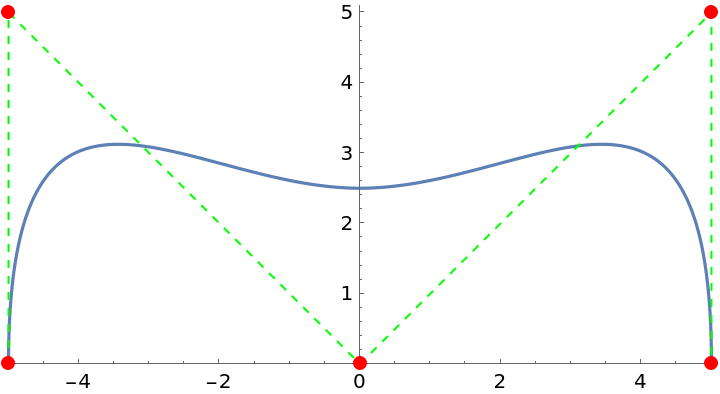The same happens when the scale factor is sufficiently large:

 In:=Out=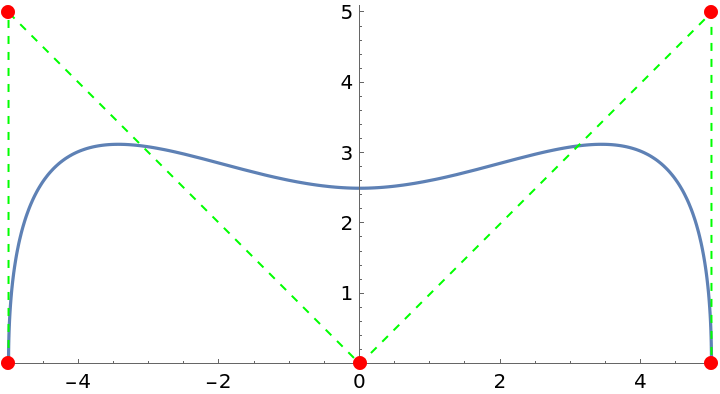Construct a parametric function from the intermediate points only (the original nodes will not be included):

 In:=Out=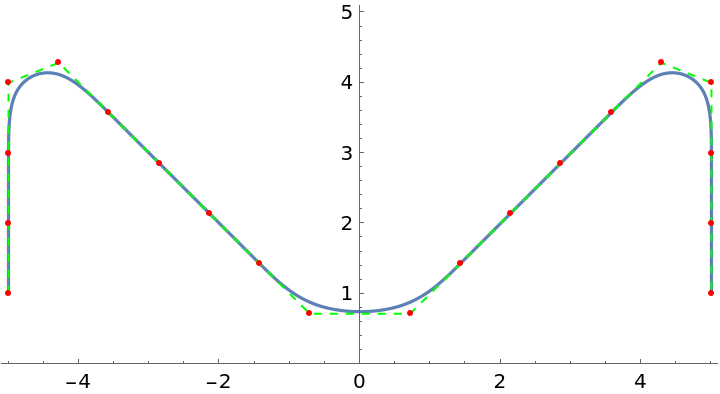### Applications (7)

Look at some jagged contours:

 In:=Out=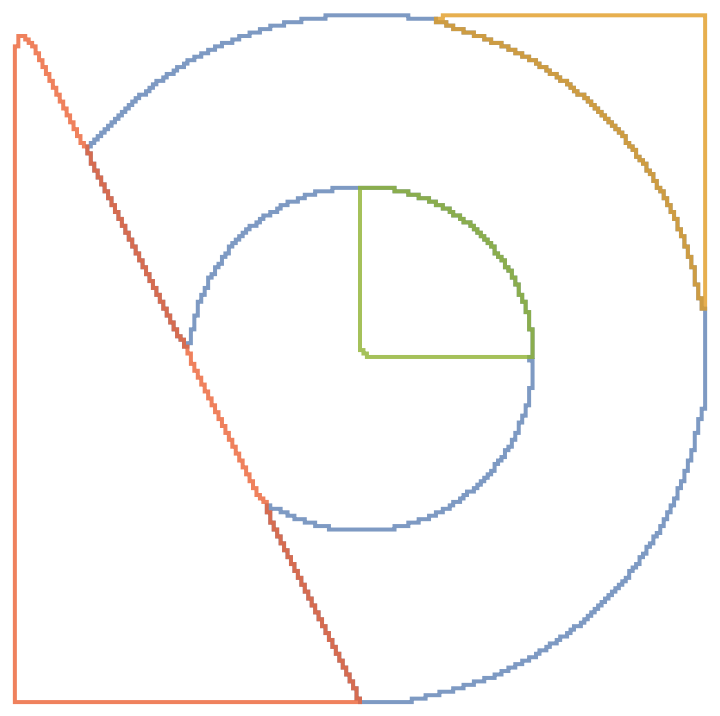Parametrize the contours and plot them:

 In:=Out=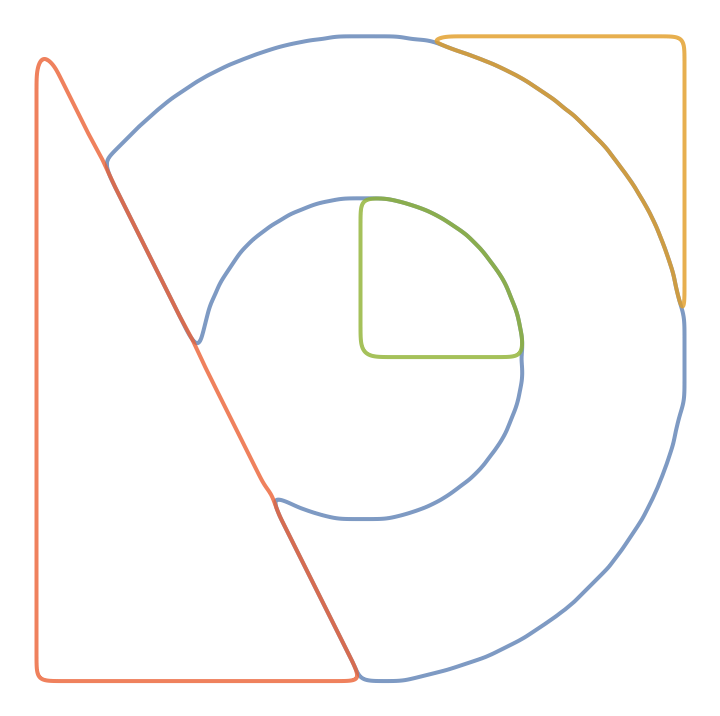With automatic node duplication, the sharp features are preserved:

 In:=Out=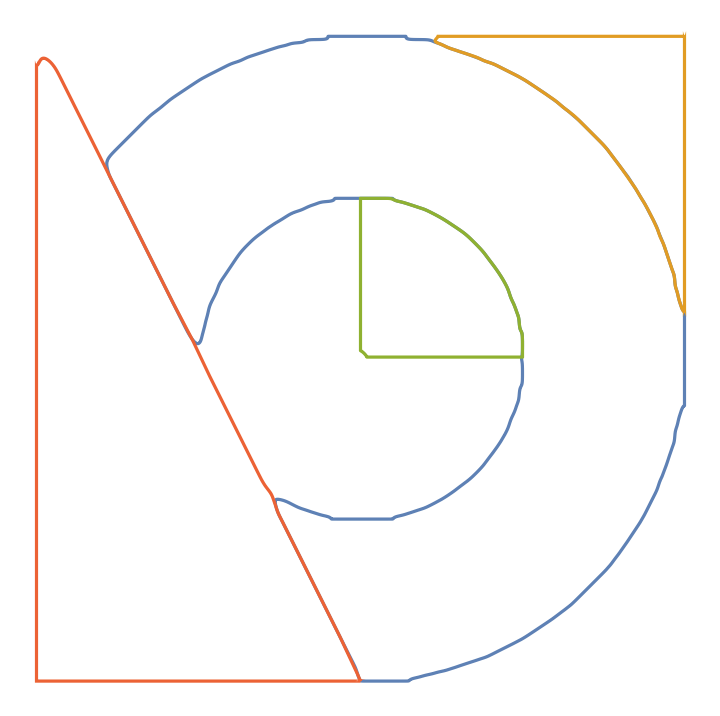Provide a custom node duplication function in order to preserve only the features you are interested in:

 In:=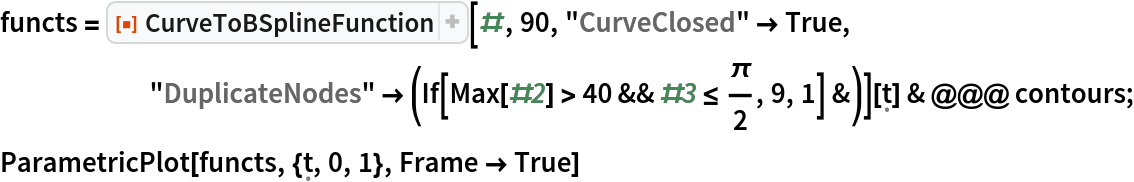Out=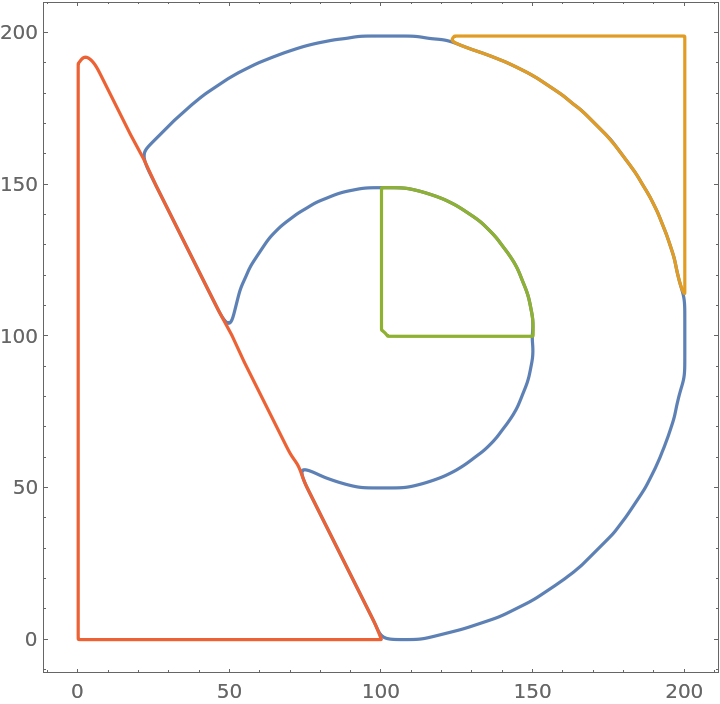Automatic weights are trying to preserve only significant sharp features:

 In:=Out=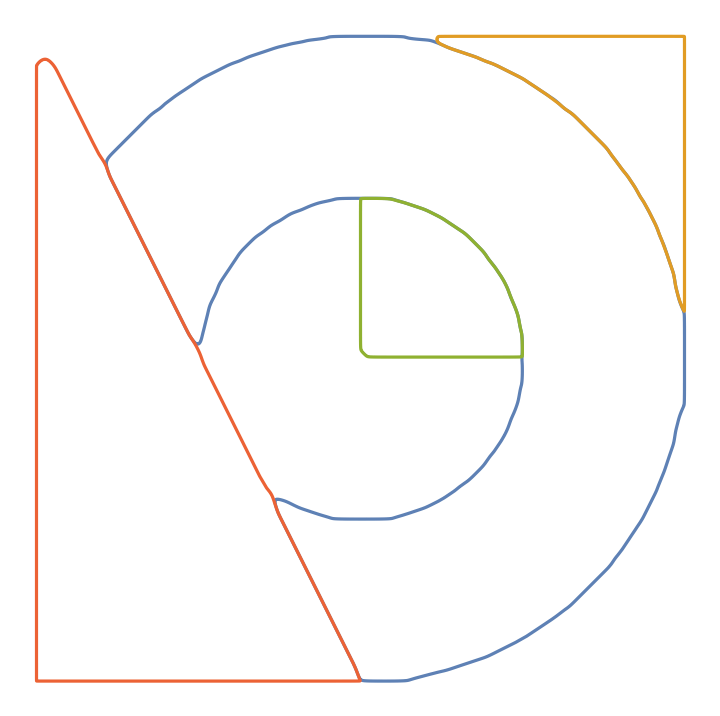Increasing the scale flattens out the features:

 In:=Out=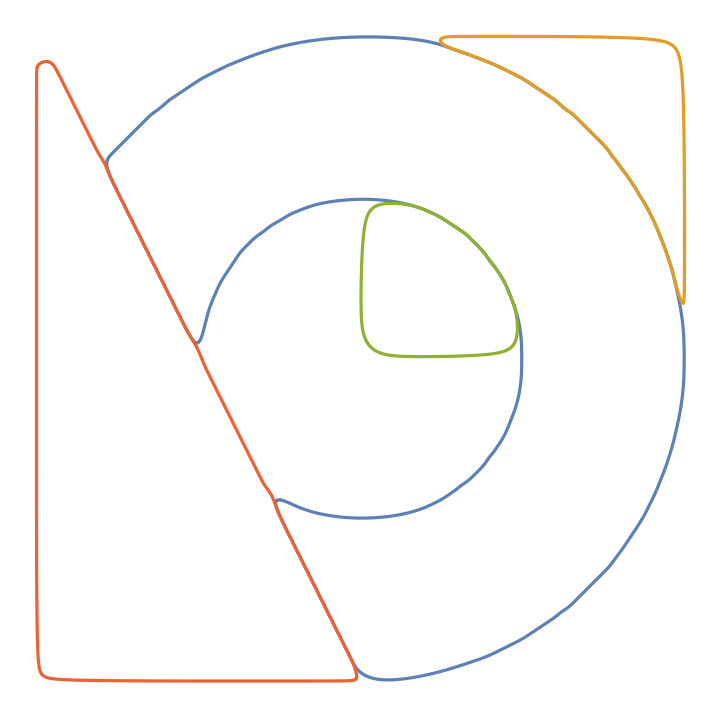Make some features in a particular region stronger by duplicating the corresponding control points:

 In:=Out=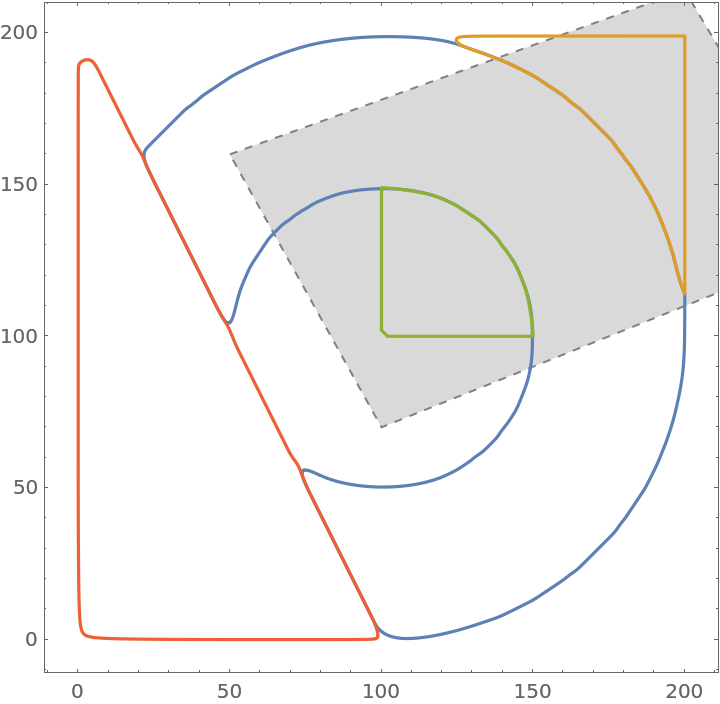### Properties and Relations (1)

Compare the areas of an exact region, its discretized version obtained through Rasterize and a smoothed version of the latter obtained via CurveToBSplineFunction:

 In:=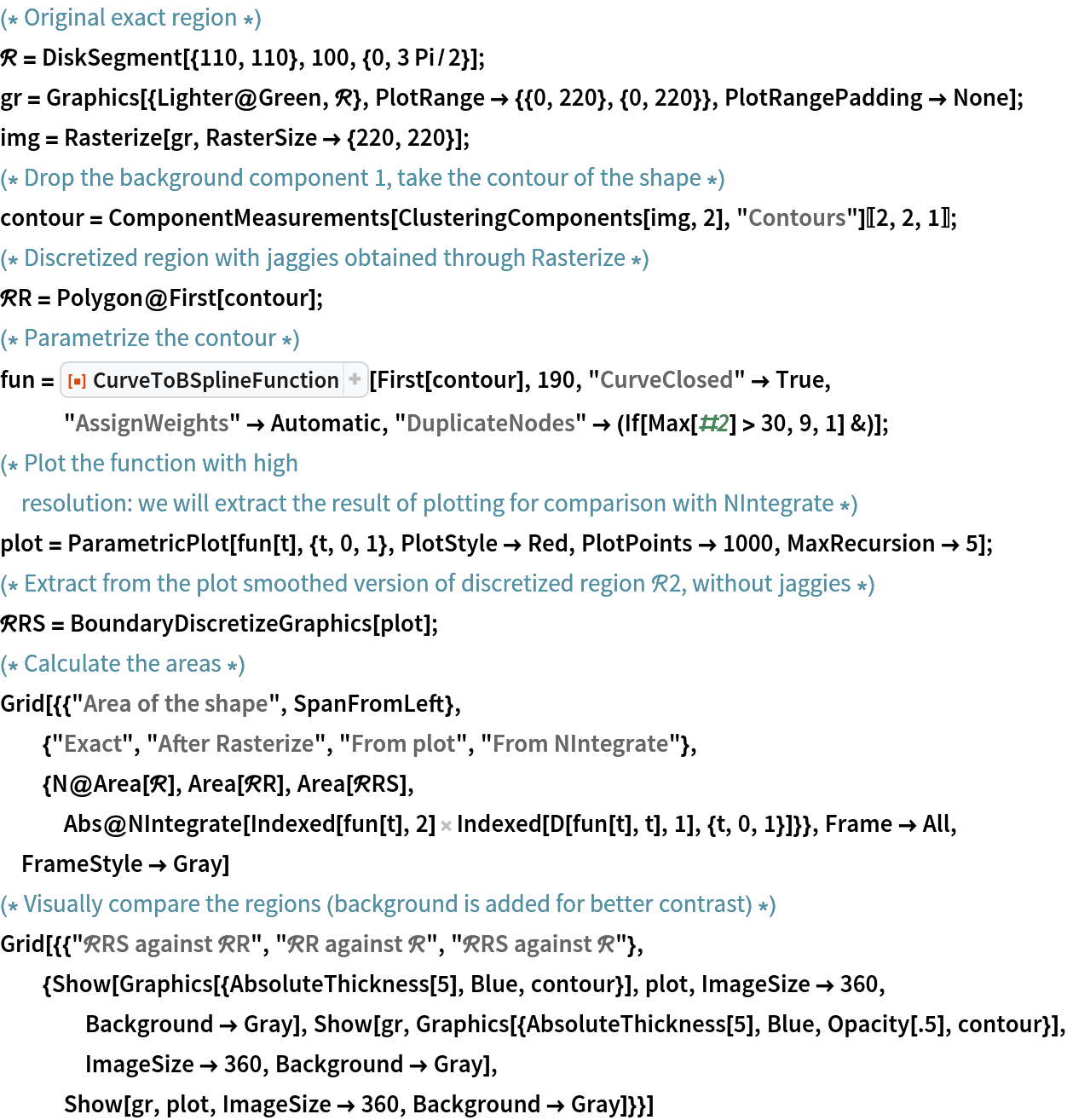Out=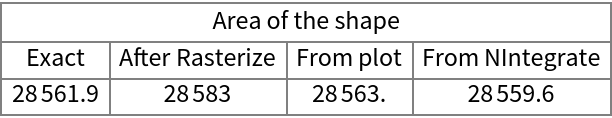Out=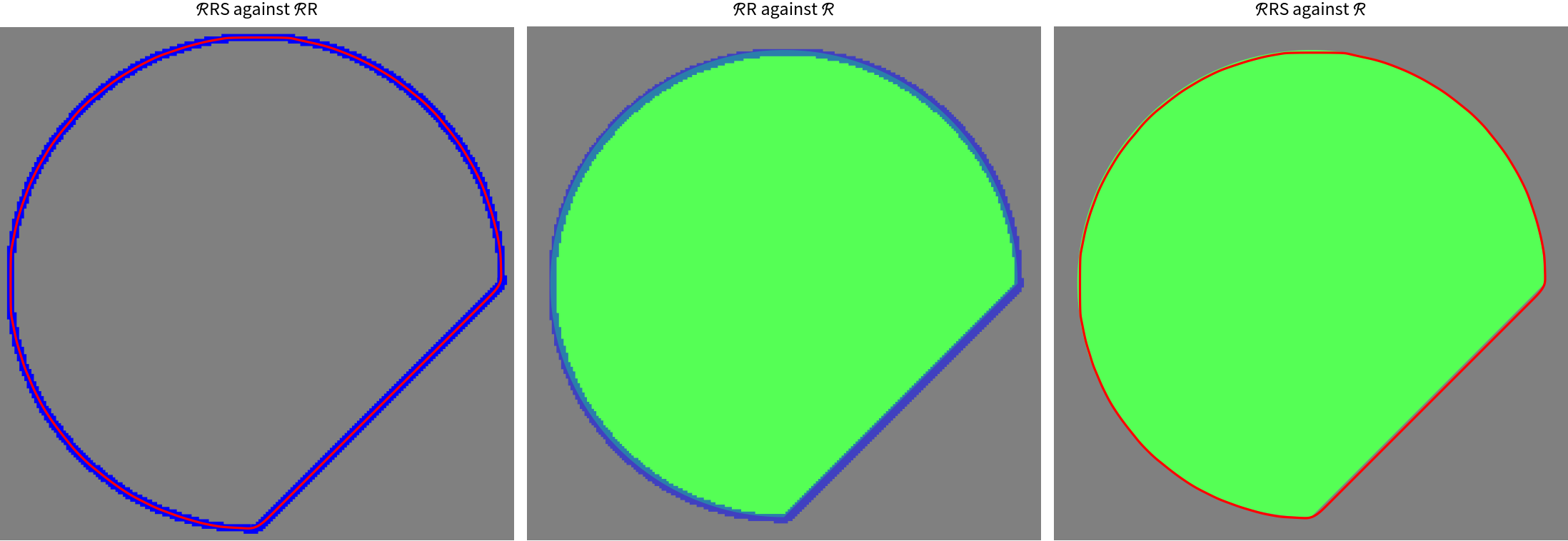### Possible Issues (2)

The default scaling factor of 1 may be insufficient to reproduce essential features of the shape:

 In:=Out=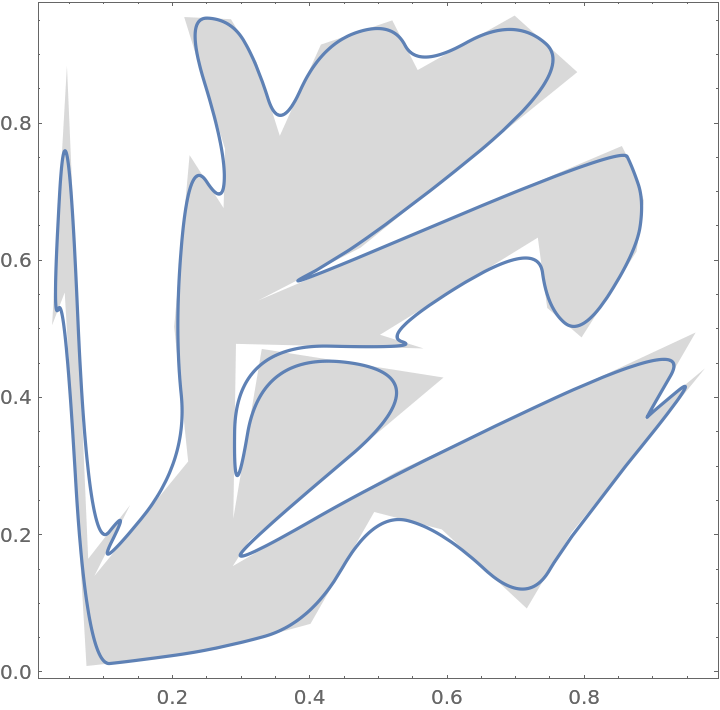Decrease the scaling factor in order to reproduce the shape more closely:

 In:=Out=Without additional intermediate control points, significant sharp features may be lost:

 In:=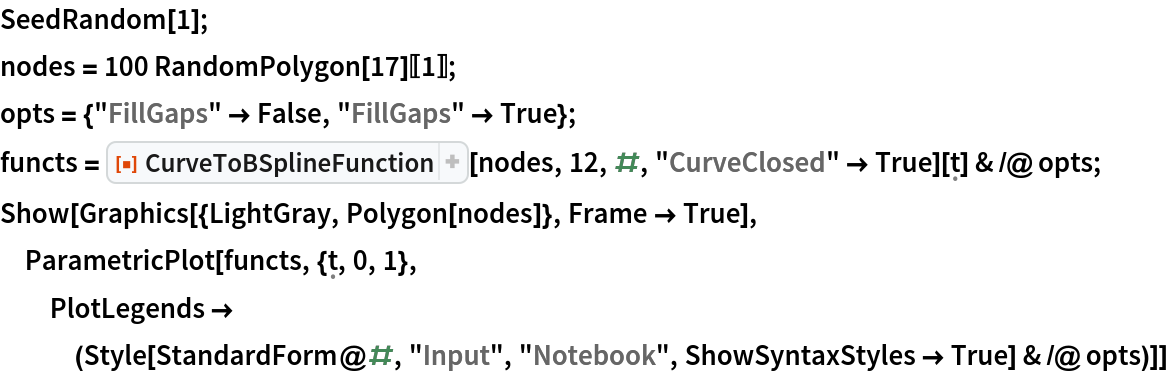Out=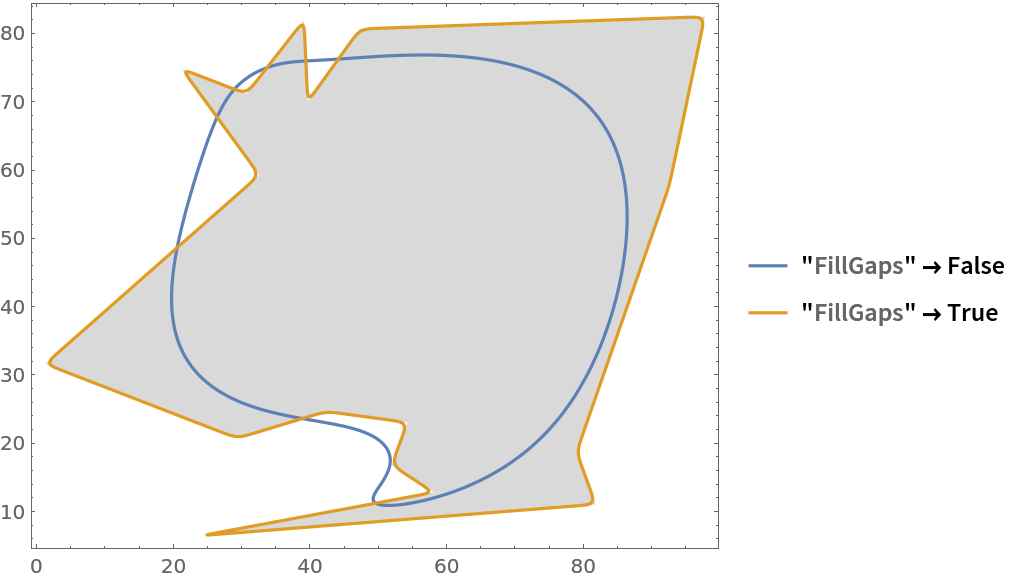### Neat Examples (2)

Create a parametric function from a discretized implicit region:

 In:=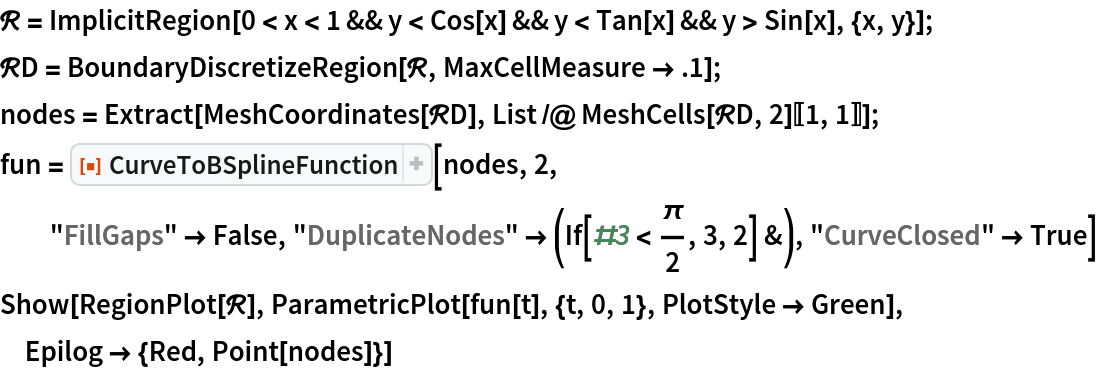Out=Out=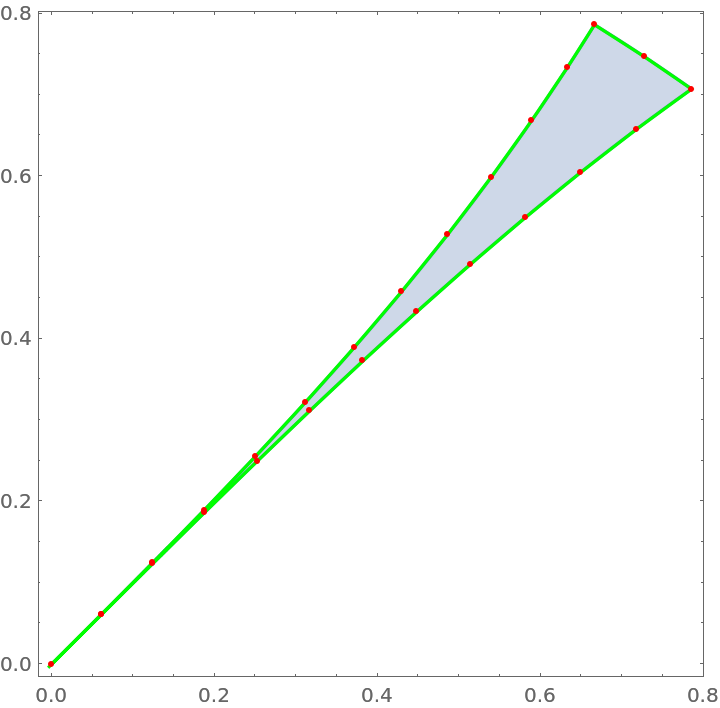Compare the areas of the exact region, its discretized version obtained through BoundaryDiscretizeRegion and a smoothed version of the latter obtained via CurveToBSplineFunction:

 In:=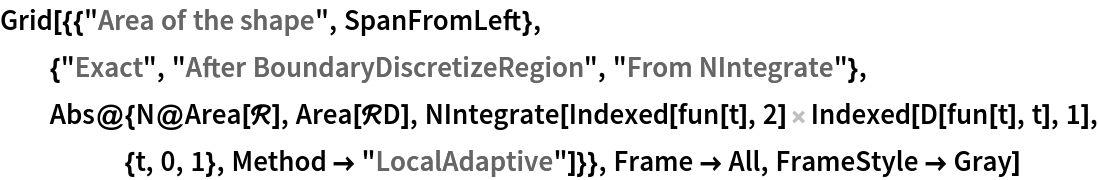Out=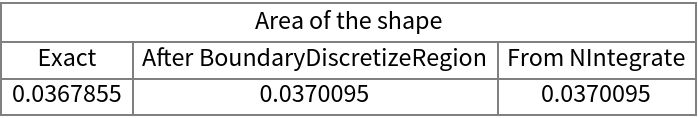AlexeyPopkov

## Version History

• 1.0.2 – 28 October 2021